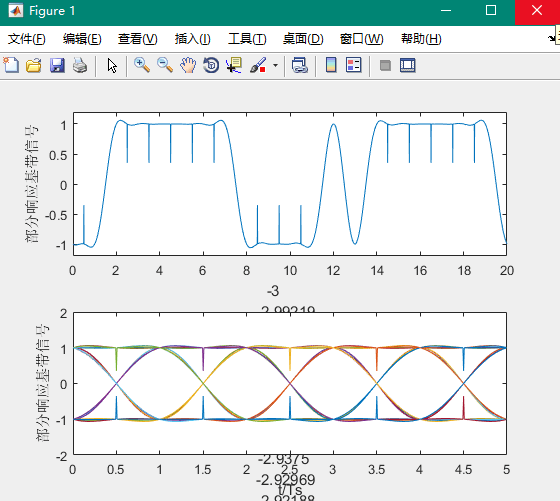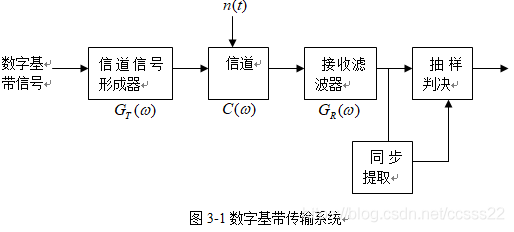• 用Matlab实现一个双音多频信号解码器。 要求： 1、输入为电话拨号按键值1、2、#等，生成对应的DTMF信号并播放； 2、按一定的SNRdB加噪声，采用FFT算法进行自动判断，输出为该键值对应的DFT变换图谱及判断得到的按...
• 用MATLAB 绘制极性非归零信号的时域波形和功率谱m文件。
• SPWM驱动H桥极性调制波形图，加深对H桥的理解。逆变开关电源工程实践。H桥的工作原理如下，正半波时Q1,Q4导通，Q2,Q3截止；负半波时Q2,Q3导通，Q1,Q4截止。而且要保证Q3和Q4,Q1和Q2不能同时导通。即Q3与Q4，Q1与Q2...
• DoublePulseGenerator，LTspice脉冲波形发生器。很方便的定义2个脉冲宽度以及两个脉冲之间的时间间隔，还可以自定义脉冲的个数。
• 2、语音信号的频谱分析在Matlab中，可以利用函数fft对信号进行快速傅立叶变换，得到信号的频谱特性，要求学生首先画出语音信号的时域波形，然后对语音信号进行频谱分析。 3、设计数字滤波器和画出其频率响应给出各...
• 极性非归零码基带信号波形及其眼图。
双极性非归零码基带信号波形及其眼图程序代代码：
clear all;
close all;
Ts = 1;
N_sample = 128;
eye_num = 5;
alpha = 1;
N_data = 10000;

dt = Ts/N_sample;
t = -3*Ts:dt:3*Ts;

%产生双极性数字信号
d = sign(randn(1,N_data));
dd = sigexpand(d,N_sample);
%基带系统冲击响应
ht = sinc(t/Ts).*(cos(alpha*pi*t/Ts))./(1-4*alpha^2 * t.^2/Ts^2+eps);
st = conv(dd,ht);
tt = -3*Ts:dt:(N_data+3)*N_sample*dt-dt;

figure(1)
subplot(211)
plot(tt,st);
axis([0 20 -1.2 1.2]);xlabel('t/Ts');ylabel('基带信号');
subplot(212)
%画眼图
ss = zeros(1,eye_num*N_sample);
ttt = 0:dt:eye_num*N_sample*dt-dt;
for k = 3:50
ss = st(k*N_sample+1:(k+eye_num)*N_sample);
drawnow;
plot(ttt,ss);hold on;
end
xlabel('t/Ts');ylabel('基带信号眼图')

需要注意的是，程序中用到sigexpand，因此要重建一个m文件，命名为：sigexpand.m 代码如下：
在这里插入代码片% sigexpand.m
%
% Simulation program to expand the symbol sequence
%
% Programmed by Y.S. Cui and H.Harada
%
function [out]=sigexpand(d,M)
N=length(d);%基带信号码元长度
out=zeros(M,N);%矩阵M为采样点  N为基带信号码元数量
out(1,:)=d;%将零矩阵第一行换成基带信号中的8个码元
out=reshape(out,1,M*N);% 1行 m*n 列

运行结果：(这个图也太可爱了吧❥）展开全文MATLAB
• 双边带抑制载波传输 (DSB-SC)： 传输其中： (a) 幅度调制产生的频率在载波频率上下对称分布，并且(b) 载波电平降低到最低实际电平，理想情况下完全抑制。 在双边带抑制载波传输（DSB-SC）调制中，与AM不同，不传输...matlab
• 通过matlab仿真，产生单极性基带信号极性基带信号，并画出其时域波形图以及功率谱，分析传输特性
• 使用stm32f103RCT6产生±5V波形，脉宽，幅度，占空比，频率皆可调。工程代码，keil5编写
• 读取通道波形并绘制波形图 import wave import matplotlib.pyplot as plt import numpy as np """读取通道波形并绘制波形图""" # 打开WAV音频 f = wave.open("./audio/audio.wav", "rb") # 读取格式信息 # ...
读取双通道波形并绘制波形图
import wave
import matplotlib.pyplot as plt
import numpy as np
"""读取双通道波形并绘制波形图"""
# 打开WAV音频
f = wave.open("./audio/audio.wav", "rb")

# 读取格式信息
# (声道数、量化位数、采样频率、采样点数、压缩类型、压缩类型的描述)
# (nchannels, sampwidth, framerate, nframes, comptype, compname)
params = f.getparams()
nchannels, sampwidth, framerate, nframes = params[:4]
print("声道数---", nchannels)
print("量化位数---", sampwidth)
print("采样频率---", framerate)
print("采样点数---", nframes)

# 读取nframes个数据，返回字符串格式
f.close()

# 将字符串转换为数组，得到一维的short类型的数组
wave_data = np.fromstring(str_data, dtype=np.short)

# 赋值的归一化
wave_data = wave_data * 1.0 / (max(abs(wave_data)))

# 整合左声道和右声道的数据
wave_data = np.reshape(wave_data, [nframes, nchannels])
# wave_data.shape = (-1, 2)   # -1的意思就是没有指定,根据另一个维度的数量进行分割

# 最后通过采样点数和取样频率计算出每个取样的时间
time = np.arange(0, nframes) * (1.0 / framerate)

plt.figure()
# 左声道波形
plt.subplot(3, 1, 1)
plt.plot(time, wave_data[:, 0])
plt.xlabel("time (seconds)")
plt.ylabel("Amplitude")
plt.title("Left channel")
plt.grid()  # 标尺

plt.subplot(3, 1, 3)
# 右声道波形
plt.plot(time, wave_data[:, 1], c="g")
plt.xlabel("time (seconds)")
plt.ylabel("Amplitude")
plt.title("Left channel")
plt.title("right channel")
plt.grid()

plt.show()

这种方法读取的是字符型，需要经过 np.fromstring(str_data, dtype=np.short)类型转换。
第二种读取wav文件的方式：
from scipy.io import wavfile
这里读取的audio直接是数组形式。
import wave
import matplotlib.pyplot as plt
import numpy as np
from scipy.io import wavfile
"""读取双通道波形并绘制波形图"""
nframes = len(f)  #获取采样点数
nchannels = 2  #  通道数=2
framerate = f #  获取采样频率
wave_data = f  #  获取音频数据
wave_data = np.reshape(wave_data, [nframes, nchannels])
# 最后通过采样点数和取样频率计算出每个取样的时间
time = np.arange(0, nframes) * (1.0 / framerate)

plt.figure()
# 左声道波形
plt.subplot(3, 1, 1)
plt.plot(time, wave_data[:, 0])
plt.xlabel("time (seconds)")
plt.ylabel("Amplitude")
plt.title("Left channel")
plt.grid()  # 标尺

plt.subplot(3, 1, 3)
# 右声道波形
plt.plot(time, wave_data[:, 1], c="g")
plt.xlabel("time (seconds)")
plt.ylabel("Amplitude")
plt.title("Left channel")
plt.title("right channel")
plt.grid()
plt.show()

结果如下：音频信号的短时频域处理
语音信号处理中，信号再频域或其他变换域上的分析处理非常重要，再频域上研究语音可以使信号在时域上无法表现出来的特征变得十分明显，一个音频信号的本质是由其频率内容来决定的。—短时傅里叶变换
import numpy as np
from scipy.io import wavfile
import matplotlib.pyplot as plt

sampling_freq, audio = wavfile.read("./audio/audio.wav")  # 读取文件

audio = audio / np.max(audio)  # 归一化，标准化

# 应用傅里叶变换
fft_signal = np.fft.fft(audio)
print(fft_signal)

fft_signal = abs(fft_signal)
print(fft_signal)

# 建立时间轴
Freq = np.arange(0, len(fft_signal))

# 绘制语音信号的
plt.figure()
plt.plot(Freq, fft_signal, color='blue')
plt.xlabel('Freq (in kHz)')
plt.ylabel('Amplitude')
plt.show()显示双通道语音信号和频谱图：
import wave
import matplotlib.pyplot as plt
import numpy as np
from scipy.io import wavfile
"""读取双通道波形并绘制波形图"""

nframes = len(f)  #获取采样点数
nchannels = 2  #  通道数=2
framerate = sampling_freq #  获取采样频率
wave_data = f  #  获取音频数据
wave_data = np.reshape(wave_data, [nframes, nchannels])
# 最后通过采样点数和取样频率计算出每个取样的时间
time = np.arange(0, nframes) * (1.0 / framerate)
#  归一化标准化
f = f / np.max(f)
# 应用傅里叶变换
f = np.fft.fft(f)
f = abs(f)
# 建立时间轴
freq = np.arange(0, len(f))

plt.figure()
# 左声道波形
plt.subplot(3, 1, 1)
plt.plot(time, wave_data[:, 0])
plt.xlabel("time (seconds)")
plt.ylabel("Amplitude")
plt.title("Left channel")
plt.grid()  # 标尺

# 右声道波形
plt.subplot(3, 1, 2)
plt.plot(time, wave_data[:, 1], c="g")
plt.xlabel("time (seconds)")
plt.ylabel("Amplitude")
plt.title("Left channel")
plt.title("right channel")
plt.grid()

# 绘制频谱图
plt.subplot(3, 1, 3)
plt.plot(freq, f, color='blue')
plt.xlabel('Freq (in kHz)')
plt.ylabel('Amplitude')
plt.grid()

plt.show()写入wav文件（合成wav文件）
先读取一个存在的音频文件，然后写入一个新的文件。
import wave
import numpy as np
import struct

f = wave.open("./audio/audio.wav", "rb")  # 已存在的wav文件
params = f.getparams()  # 读取该文件的参数
# 读取原文件的通道数，量化位数，采样频率，采样点数
nchannels_old, sampwidth_old, framerate_old, nframes_old = params[:4]
#转换读取的格式
waveData = np.fromstring(strData, dtype=np.int16)
f.close()
waveData = waveData * 1.0 / (max(abs(waveData)))

# wav文件写入
# 待写入wav的数据，这里仍然取waveData数据
outData = waveData
outwave = wave.open("./audio/compose_test.wav", 'wb')
# 通道数，量化位数，采样频率，采样点数设置。也可自行选择
nchannels = nchannels_old
sampwidth = sampwidth_old
framerate = framerate_old
nframes = nframes_old
# 写入文件参数配置
comptype = "NONE"
compname = "not compressed"
outwave.setparams((nchannels, sampwidth, framerate, nframes,
comptype, compname))

for i in outData:
outwave.writeframes(struct.pack('h', int(i * 64000 / 2)))

# struct.pack(FMT, V1)将V1的值转换为FMT格式字符串
outwave.close()

录音
以SAMPLING_RATE为采样频率，每次读入一块有NUM_SAMPLES个采样的数据块，当读入的采样数据中有COUNT_NUM个值大于LEVEL的取样的时候，将数据保存进WAV文件，一旦开始保存数据，所保存的数据长度最短为SAVE_LENGTH个块。WAV文件以保存时的时刻作为文件名。
从声卡读入的数据和从WAV文件读入的类似，都是二进制数据，由于我们用paInt16格式(16bit的short类型)保存采样值，因此将它自己转换为dtype为np.short的数组。
'''
以SAMPLING_RATE为采样频率，
每次读入一块有NUM_SAMPLES个采样点的数据块，
当读入的采样数据中有COUNT_NUM个值大于LEVEL的取样的时候，
将采样数据保存进WAV文件，
一旦开始保存数据，所保存的数据长度最短为SAVE_LENGTH个数据块。

从声卡读入的数据和从WAV文件读入的类似，都是二进制数据，
由于我们用paInt16格式(16bit的short类型)保存采样值，
因此将它自己转换为dtype为np.short的数组。
'''

from pyaudio import PyAudio, paInt16
import numpy as np
import wave

# 将data中的数据保存到名为filename的WAV文件中
def save_wave_file(filename, data):
wf = wave.open(filename, 'wb')
wf.setnchannels(1)          # 单通道
wf.setsampwidth(2)          # 量化位数
wf.setframerate(SAMPLING_RATE)  # 设置采样频率
wf.writeframes(b"".join(data))  # 写入语音帧
wf.close()

NUM_SAMPLES = 2000      # pyAudio内部缓存块的大小
SAMPLING_RATE = 8000    # 取样频率
LEVEL = 1500           # 声音保存的阈值，小于这个阈值不录
COUNT_NUM = 20 # 缓存快类如果有20个大于阈值的取样则记录声音
SAVE_LENGTH = 8 # 声音记录的最小长度：SAVE_LENGTH * NUM_SAMPLES 个取样

# 开启声音输入
pa = PyAudio()
stream = pa.open(format=paInt16, channels=1, rate=SAMPLING_RATE, input=True,
frames_per_buffer=NUM_SAMPLES)

save_count = 0  # 用来计数
save_buffer = []    #

while True:
# 读入NUM_SAMPLES个取样
# 将读入的数据转换为数组
audio_data = np.fromstring(string_audio_data, dtype=np.short)
# 计算大于LEVEL的取样的个数
large_sample_count = np.sum( audio_data > LEVEL )
print(np.max(audio_data))
# 如果个数大于COUNT_NUM，则至少保存SAVE_LENGTH个块
if large_sample_count > COUNT_NUM:
save_count = SAVE_LENGTH
else:
save_count -= 1

if save_count < 0:
save_count = 0

if save_count > 0:
# 将要保存的数据存放到save_buffer中
save_buffer.append( string_audio_data )
else:
# 将save_buffer中的数据写入WAV文件，WAV文件的文件名是保存的时刻
if len(save_buffer) > 0:
filename = "recorde" + ".wav"
save_wave_file(filename, save_buffer)
print(filename, "saved")
break

MFCC特征提取
import numpy as np
import matplotlib.pyplot as plt
from scipy.io import wavfile
from python_speech_features import mfcc, logfbank

# 读取输入音频文件

# 提取MFCC和滤波器组特征
mfcc_features = mfcc(audio, sampling_freq)
filterbank_features = logfbank(audio, sampling_freq)

print('\nMFCC:\n窗口数 =', mfcc_features.shape)
print('每个特征的长度 =', mfcc_features.shape)
print('\nFilter bank:\n窗口数 =', filterbank_features.shape)
print('每个特征的长度 =', filterbank_features.shape)

# 画出特征图，将MFCC可视化。转置矩阵，使得时域是水平的
mfcc_features = mfcc_features.T
plt.matshow(mfcc_features)
plt.title('MFCC')
# 将滤波器组特征可视化。转置矩阵，使得时域是水平的
filterbank_features = filterbank_features.T
plt.matshow(filterbank_features)
plt.title('Filter bank')

plt.show()

结果如下（因为输入的音频较大，故有点长。在传统的音频处理中，输入音频大多为短时音频，形状为矩形。感兴趣的可尝试输入不同的音频）：语谱图
import wave
import matplotlib.pyplot as plt
import numpy as np

f = wave.open('./audio/audio.wav', 'rb')
params = f.getparams()
nchannels, sampwidth, framerate, nframes = params[:4]
wavedata = np.fromstring(strdata, dtype=np.int16)
wavedata = wavedata * 1.0 / (max(abs(wavedata)))
wavedata = np.reshape(wavedata, [nframes, nchannels]).T
f.close()

plt.specgram(wavedata, Fs=framerate, scale_by_freq=True, sides = 'default')
plt.ylabel('Frequency(HZ)')
plt.xlabel('Time(s)')
plt.show()语音识别
如下是一个语音识别的实例
import os
import argparse
import numpy as np
from scipy.io import wavfile
from hmmlearn import hmm
from python_speech_features import mfcc

# 解析命令行的输入参数
def build_arg_parser():
parser = argparse.ArgumentParser(description='Trains the HMM classifier')
help="Input folder containing the audio files in subfolders")
return parser

# 创建类，处理HMM相关过程
class HMMTrainer(object):
'''用到高斯隐马尔科夫模型
n_components：定义了隐藏状态的个数
cov_type：定义了转移矩阵的协方差类型
n_iter:定义了训练的迭代次数
'''
def __init__(self, model_name='GaussianHMM', n_components=4, cov_type='diag', n_iter=1000):
self.model_name = model_name
self.n_components = n_components
self.cov_type = cov_type
self.n_iter = n_iter
self.models = []

if self.model_name == 'GaussianHMM':
self.model = hmm.GaussianHMM(n_components=self.n_components,
covariance_type=self.cov_type, n_iter=self.n_iter)
else:
raise TypeError('Invalid model type')

# X是二维数组，其中每一行有13个数
def train(self, X):
np.seterr(all='ignore')
self.models.append(self.model.fit(X))

# 对输入数据运行模型
def get_score(self, input_data):
return self.model.score(input_data)

if __name__=='__main__':
# 解析输入参数
args = build_arg_parser().parse_args(['--input-folder', 'input_folder'])
input_folder = args.input_folder

hmm_models = [] # 初始化隐马尔科夫模型的变量

# 解析输入路径
for dirname in os.listdir(input_folder):
# 获取子文件夹名称
subfolder = os.path.join(input_folder, dirname)
if not os.path.isdir(subfolder):
continue
# 子文件夹名称即为该类的标记
# 提取特征
label = subfolder[subfolder.rfind('/') + 1:]

# 初始化变量
X = np.array([])
y_words = []

# 迭代所有音频文件(分别保留一个进行测试)
for filename in [x for x in os.listdir(subfolder) if x.endswith('.wav')][:-1]:
# 读取每个音频文件
filepath = os.path.join(subfolder, filename)

# 提取MFCC特征
mfcc_features = mfcc(audio, sampling_freq)

# 将MFCC特征添加到X变量
if len(X) == 0:
X = mfcc_features
else:
X = np.append(X, mfcc_features, axis=0)

# 添加标记
y_words.append(label)

print('X.shape =', X.shape)
# 训练并且保存HMM模型
hmm_trainer = HMMTrainer()
hmm_trainer.train(X)
hmm_models.append((hmm_trainer, label))

hmm_trainer = None

# 测试文件
input_files = [
'data/pineapple/pineapple15.wav',
'data/orange/orange15.wav',
'data/apple/apple15.wav',
'data/kiwi/kiwi15.wav'
]

# 为输入数据分类
for input_file in input_files:
# 读取每个音频文件

# 提取MFCC特征
mfcc_features = mfcc(audio, sampling_freq)

# 定义变量
max_score = 0
output_label = None
score_data = []
# 迭代HMM模型并选取得分最高的模型
for item in hmm_models:
hmm_model, label = item
score = hmm_model.get_score(mfcc_features)
score_data.append(score)
max_score = max(score_data)
for item in hmm_models:
hmm_model, label = item
score = hmm_model.get_score(mfcc_features)
if score >= max_score:
max_score = score
output_label = label

# 打印结果
print("\nTrue:", input_file[input_file.find('/')+1:input_file.rfind('/')])
print("Predicted:", output_label)信号分帧wlen为帧长，inc 为帧移，重叠部分为overlap，overlap = wlen - inc
信号帧数为：fn = (N - overlap) / inc = (N - wlen) / inc + 1
N为语音数据长度。
加窗
通常对信号截断，分帧需要加窗，因为截断都有频域能量泄露，而窗函数可以减少截断带来的影响。窗函数再scipy.signal信号处理工具箱中。
短时能量
短时能量主要用于区分浊音段和清音段
下面绘制未加窗，加窗，以及短时能量图：
import numpy as np
import wave
import matplotlib.pyplot as plt
import scipy.signal as signal

wlen = 512  # 每一帧信号的长度
inc = 128  #  帧移
f = wave.open('./data/apple/apple01.wav', 'rb')
params = f.getparams()
nchannels, sampwidth, framerate, nframes = params[:4]  # 读取文件的参数并赋值-通道数，量化位数，采样频率，采样点数
wave_data = np.fromstring(str_data, dtype=np.short)  # 处理采样点数的数据，将str型转为为short型
wave_data = wave_data * 1.0 / (max(abs(wave_data)))  #  归一化处理

time = np.arange(0, wlen) * (1.0 / framerate)

signal_length = len(wave_data)  # 获取信号长度

if signal_length <= wlen:
nf = 1
else :
nf = int(np.ceil((1.0 * signal_length - wlen + inc) / inc))

pad_length = int((nf - 1 ) * inc + wlen)
indices = np.tile(np.arange(0, wlen), (nf, 1)) + np.tile(np.arange(0, nf*inc, inc), (wlen, 1)).T

indices = np.array(indices, dtype = np.int32)
# 绘制第10-11帧的图像
a = frames[10:11]
# 使用汉明窗
windown = np.hanning(wlen)
b = a * windown
c = np.square(b)

plt.figure(figsize=(10, 4))
# 绘制未加窗的图
plt.subplot(3, 1, 1)
plt.plot(time, a, c='b')
plt.xlabel("no-window")
plt.grid()
#  绘制增加汉明窗的图
plt.subplot(3, 1, 2)
plt.plot(time, b, c='g')
plt.xlabel("hanning-window")
plt.grid()
#  绘制短时能量图
plt.subplot(3, 1, 3)
plt.plot(time, c, c='r')
plt.xlabel("short-power")
plt.grid()
plt.show()绘制第10-11帧的图像
a = frames[10:11]
使用汉明窗
windown = np.hanning(wlen) b = a * windown c = np.square(b)
plt.figure(figsize=(10, 4))
绘制未加窗的图
plt.subplot(3, 1, 1) plt.plot(time, a, c=‘b’) plt.xlabel(“no-window”) plt.grid()
绘制增加汉明窗的图
plt.subplot(3, 1, 2) plt.plot(time, b, c=‘g’) plt.xlabel(“hanning-window”) plt.grid()
绘制短时能量图
plt.subplot(3, 1, 3) plt.plot(time, c, c=‘r’) plt.xlabel(“short-power”) plt.grid() plt.show()展开全文自然语言处理
• 根据不同的分类标准可以分为周期信号和非周期信号，连续信号和离散信号，能量信号和功率信号等等。这里需要掌握的是信号的能量表达式，功率信号和能量信号的定义以及重点掌握它们的时域性质和频域性质。可参考通信...
1. 功率信号和能量信号及它们的时域和频域性质
确知信号的分类。根据不同的分类标准可以分为周期信号和非周期信号，连续信号和离散信号，能量信号和功率信号等等。这里需要掌握的是信号的能量表达式，功率信号和能量信号的定义以及重点掌握它们的时域性质和频域性质。可参考通信原理第2章内容。
分类  时域性质频域性质功率信号能量无限，功率有限一般为周期信号自相关、互相关函数频谱、功率谱密度能量信号能量有限，平均功率为0一般为非周期信号，存在傅里叶变换自相关、互相关函数频谱密度、能量谱密度
2. 窄带信号和宽带信号。
如何去界定？概念理解清楚，后面就不会糊涂。
3. 希尔伯特变换和解析信号
单边谱和双边谱。参考链接。
4. 实信号、复信号和复包络之间的关系
搞清楚实信号、复信号和复包络之间的关系。
5. 时宽和带宽以及测量的均方根误差
输入信噪比越大，测距和测速的误差就越小，精度越高。
增大信号带宽能提高测距精度，增大信号时宽能提高测速精度。这就是“雷达测不准原理”
6. 信号波形分类
连续波信号单载频（只能测速，无法测距，交通测速雷达）调频（线性、非线性）FMCW用的比较多，但是也有缺点 调相（二相编码，多相编码） 多频连续波间歇式连续波脉冲信号单载频（又称为简单脉冲）脉内，脉间或脉组间编码（相位、频率编码）相参脉冲串信号（均匀脉冲串、参差脉冲串）
7. 模糊函数和分辨率
模糊函数的定义以及性质。
展开全文模糊函数
• 用MATLAB绘制波形形成后的极性非归零码时域波形及其眼图的绘制需要注意的是，程序中用到sigexpand，因此要重建一个m文件，命名为：sigexpand.m。
• 一、简介 主要研究了数字信号的基带传输的基本概念及数字...之后介绍了数字基带信号的最佳接收的条件以及如何通过示波器观察基带信号波形。最后按照仿真过程基本步骤用MATLAB的仿真工具实现了数字基带传输系统的仿
一、获取代码方式
获取代码方式1： 完整代码已上传我的资源：【数字基带信号】基于matlab数字基带信号波形仿真【含Matlab源码 988期】
获取代码方式2： 通过订阅紫极神光博客付费专栏，凭支付凭证，私信博主，可获得此代码。
备注： 订阅紫极神光博客付费专栏，可免费获得2份代码（有效期为订阅日起，三天内有效）；
二、数字基带信号波形仿真简介
主要研究了数字信号的基带传输的基本概念及数字信号基带传输的传输过程和如何用MATLAB软件仿真设计数字基带传输系统。本文首先介绍了MATLAB仿真软件。然后介绍了本课题的理论依据，包括数字通信，数字基带传输系统的组成及数字基带信号的传输过程。接着介绍了数字基带传输系统的特性包括数字PAM信号功率普密度及常用线路码型，并通过比较最终选择双极性不归零码。之后介绍了数字基带信号的最佳接收的条件以及如何通过示波器观察基带信号的波形。最后按照仿真过程基本步骤用MATLAB的仿真工具实现了数字基带传输系统的仿真过程，对系统进行了分析。
数字基带传输系统在实际数字通信系统中的应用虽然没有频带传输应用广泛，但仍有相当多的应用范围。而且最为重要的是数字基带传输系统的基本理论不仅适用于数字基带传输系统，而且还适用于频带传输，因为所有窄的带通信号、线性带通系统及等效低通系统都对等效低通信号的响应均可用其等效低通信号、等效低通系统及等效低通系统对等效低通信号的响应来表示，因而频带传输系统可通过它的等效低通（或等效基带）传输系统的理论分析及计算机仿真来研究它的性能，因而掌握数字基带传输的基本理论十分重要，它在数字通信系统中具有普遍意义。
1 基带传输系统简介 如果数字调制器的载波是周期性的脉冲，用数字序列去调制脉冲载波的某参数，则可将数字序列转换成为相应的信号波形，这就被称为数字脉冲调制器。而数字脉冲调制器输出信号波形的功率谱密度是低通型的，所占频带是从直流或低频开始的，其带宽是有限的。那么就称此数字信号为数字基带信号。若通信信道的传递函数是低通型的，则称此信道为基带信道，又称基带信道为低通信道，如同轴电缆和双绞线有线信道均属基带信道。数字基带信号通过基带信道进行传输，则称此传输系统为数字基带传输系统。2 基带传输系统结构图
基带传输系统主要由信道信号形成器、信道、接收滤波器和抽样判决器组成。为了保证系统可靠有序地工作，还应有同步系统。 信道信号形成器：把原始基带信号变换成适合于信道传输的基带信号，这种变换主要是通过码型变换和波形变换来实现的，其目的是与信道匹配，便于传输，减小码间串扰，利于同步提取和抽样判决。 信道：允许基带信号通过的媒质。信道的传输特性通常不满足无失真传输条件，甚至是随机变化的。另外信道还会进入噪声。在通信系统的分析中，常常把噪声n（t）等效，集中在信道中引入。 接收滤波器：滤除带外噪声，对信道特性均衡，使输出的基带波形有利于抽样判决。 抽样判决器：在传输特性不理想及噪声背景下，在规定的时刻（由位定时脉冲控制）对接收滤波器的输出波形进行抽样判决，以恢复或再生基带信号。而用来抽样的位定时脉冲则依靠同步提取电路从接收信号中提取，位定时的准确与否将直接影响判决效果。
3 基带传输过程
终端设备编码器所产生的脉冲序列将作为为基带传输系统的输入信号，为了使这种脉冲序列能在信道中进行传输，一般要通过码型变换器将二进制脉冲序列变为双极性码（AMI码或HDB3码），有时为了使信号在基带传输系统内的码间干扰降到最低，还要进行波形变换。由于信道特性不理想或者噪声的干扰，会使经过信道的信号受到干扰而变形。在接收端为了减小噪声的影响，首先会把通过信道的信号引入接收滤波器，然后再经过均衡器，校正由于信道特性（包括接收滤波器在内）不理想而产生的波形失真或码间串扰。最后在取样定时脉冲到来时，进行判决以恢复基带数字码脉冲。
三、部分源代码
close all
clear all
%采样点数的设置
k=14;
%每码元采样数的设置
L= 32;
N=2^k;
M=N/L;%M为码元个数
dt=1/L;%时域采样间隔
T=N*dt;%时域截断区间
df=1.0/T;%频域采样间隔
Bs=N*df/2;%频域截断区间
t=linspace(-T/2,T/2,N);%产生时域采样点
f=linspace(-Bs,Bs,N);%产生频域采样点
EP1=zeros(size(f));
EP2=zeros(size(f));
EP3=zeros(size(f));

%程序第1部分：随机产生1000列0、1信号序列，分别对其进行双极性归零编码和不归零%编码，并且求各自的功率谱密度，求功率谱密度的均值
for x=1:1000%取1000次样值
a=round(rand(1,M));%产生一个长度为M的随机序列a，0和1等概出现
nrz=zeros(L,M);%产生一个L行M列的nrz矩阵，初始化为全0矩阵
rz=zeros(L,M);%产生一个L行M列的rz矩阵，初始化为全0矩阵
for i=1:M
if a(i)==1
nrz(:,i)=1;%使nrz矩阵第i列全部元素都为1
rz(1:L/2,i)=1;%使rz矩阵第i列前L/2个元素为1
else
nrz(:,i)=0;%使nrz矩阵第i列全部元素都为-1
rz(1:L/2,i)=0;%使rz矩阵第i列前L/2个元素为-1
end
end
%分别重排nrz、rz矩阵为1行N列的矩阵
nrz=reshape(nrz,1,N);
rz=reshape(rz,1,N);
%做傅里叶变换并算出功率谱密度
NRZ=t2f(nrz,dt);
P1=NRZ.*conj(NRZ)/T;
RZ=t2f(rz,dt);
P2=RZ.*conj(RZ)/T;
%求功率谱密度的均值
EP1=(EP1*(x-1)+P1)/x;
EP2=(EP2*(x-1)+P2)/x;
end
%采样点数的设置
k=14;
%每码元采样数的设置
L= 32;
N=2^k;
M=N/L;%M为码元个数
dt=1/L;%时域采样间隔
T=N*dt;%时域截断区间
df=1.0/T;%频域采样间隔
Bs=N*df/2;%频域截断区间
t=linspace(-T/2,T/2,N);%产生时域采样点
f=linspace(-Bs,Bs,N);%产生频域采样点
EP1=zeros(size(f));
EP2=zeros(size(f));
EP3=zeros(size(f));

%程序第2部分：随机产生1000列0、1信号序列，分别对其进行双极性归零编码和不归零%编码，并且求各自的功率谱密度，求功率谱密度的均值
for x=1:1000%取1000次样值
a=round(rand(1,M));%产生一个长度为M的随机序列a，0和1等概出现
nrz=zeros(L,M);%产生一个L行M列的nrz矩阵，初始化为全0矩阵
rz=zeros(L,M);%产生一个L行M列的rz矩阵，初始化为全0矩阵
for i=1:M
if a(i)==1
nrz(:,i)=1;%使nrz矩阵第i列全部元素都为1
rz(1:L/2,i)=1;%使rz矩阵第i列前L/2个元素为1
else
nrz(:,i)=-1;%使nrz矩阵第i列全部元素都为-1
rz(1:L/2,i)=-1;%使rz矩阵第i列前L/2个元素为-1
end
end

%分别重排nrz、rz矩阵为1行N列的矩阵
nrz=reshape(nrz,1,N);
rz=reshape(rz,1,N);
%做傅里叶变换并算出功率谱密度
NRZ=t2f(nrz,dt);
P1=NRZ.*conj(NRZ)/T;
RZ=t2f(rz,dt);
P2=RZ.*conj(RZ)/T;
%求功率谱密度的均值
EP1=(EP1*(x-1)+P1)/x;
EP2=(EP2*(x-1)+P2)/x;
end


四、运行结果五、matlab版本及参考文献
1 matlab版本 2014a
2 参考文献  沈再阳.精通MATLAB信号处理[M].清华大学出版社，2015. 高宝建,彭进业,王琳,潘建寿.信号与系统——使用MATLAB分析与实现[M].清华大学出版社，2020. 王文光,魏少明,任欣.信号处理与系统分析的MATLAB实现[M].电子工业出版社，2018.
展开全文matlab
• 双音多频信号（Dual-Tone Multi-Frequency, DTMF），电话系统中电话机与交换机之间的一种用户信令，通常用于发送被叫号码。 在使用双音多频信号之前，电话系统中使用一连串的断续脉冲来传送被叫号码，称为脉冲...
• MATLAB 语音信号处理之显示频谱、滤波显示原始语音信号的特征频带（谱峰位置）设计低通滤波器对语音信号进行处理 显示原始语音信号的特征频带（谱峰位置） 利用Windows自带的录音机录制了一个16s的语音文件，编写...matlab 语音识别
• 文章主要分为三大部分信号发生器设置脉冲的基本原理信号发生器设置脉冲波形的详细操作流程示波器观测脉冲波形的基本设置一. 信号发生器设置脉冲波形的基本原理图 1 信号发生器简图如图1所示，为信号发生器的...
• 在无线电通信中，连续波射频信号（正弦载波）在传输之前由音频波形调制其幅度。 音频波形修改载波的幅度并确定波形的包络。 在频域中，幅度调制产生的信号功率集中在载波频率和两个相邻的边带上。 每个边带的带宽与...matlab
• ## Matlab：语音信号处理与滤波

万次阅读 多人点赞 2019-06-12 13:53:37
文章目录语音信号的采集对语音信号进行频谱分析设计数字滤波器利用滤波器进行滤波 语音信号的采集 首先利用win自带的录音机（没有的话手机录也行...处理语音信号的时域波形图 对语音信号进行快速傅立叶变换，得到信...Matlab
• 用MATLAB 绘制极性非归零信号的时域波形和功率谱。MATLAB
• 这是用于求语音信号时域波形自相关函数的代码，希望会有所帮助。自相关函数
• 本文目的是使用STM32的DAC（数模转换）实现音频信号波形的输出。这里分为两个部分：第一部分实现特定频率单音频信号波形的输出；第二部分实现歌曲音频信号波形的输出。 目录（一）DAC简介1.STM32的DAC2.DAC主要特征...stm32 嵌入式
• 一、简介 1．课程设计的目的 通过数字信号处理的课程设计，使学生对信号的采集，处理，传输，...画出采样后语音信号的时域波形和频谱图；给定滤波器的性能指标，采用窗函数法和双线性变换设计滤波器，并画出滤波器的频matlab 语音识别
• ## MATLAB语音信号处理

千次阅读 多人点赞 2019-10-05 07:12:34
数字信号处理课设，我们使用MATLAB对语音信号进行了一系列处理，并将其所有功能集中于下图界面中： 这个界面涉及功能众多，其中包括语音信号的观察分析、音色变换、AM调制解调、减抽样、加噪去噪、相频分析和幅频...
• 声音）是模拟信号，现实生活中表现为连续的、平滑的波形，其横坐标为时间轴，纵坐标表示声音的强弱。 我们需要将其保存为数字信号再进行处理。 1. 声音三要素 1.1 音调 人耳对声音高低的感觉称为音调。音调主要...python
• 摘要：MATLAB是十分强大的用于数据分析和处理的工程实用软件,利用其来进行语音信号的分析、处理和可视化十分便捷。文中介绍了在MATLAB环境中如何驱动声卡采集语音信号和语音信号采集后的文档处理方法,并介绍了FFT...
• 通信原理实验，附有实验报告，实验主要内容如下： 1、通过MATLAB产生一串信码...2、对产生的信码序列分别进行单、极性NRZ和RZ波形的成形，即编程实现; 3、产生1000个随机信号序列，画出两种波形的平均功率谱密度曲线matlab
• 摘要利用所学习的数字信号处理知识，设计了一个有趣的音效处理系统，首先设计了几种不同的滤波器对声音进行滤波处理，分析了时域和频域的变化，比较了经过滤波处理后的声音与原来的声音有何变化。同时设计实现了语音...matlab 数学建模 语音识别
• 2.画出采样后的语音信号的时域波形和频谱图； 3.给定滤波器的性能指标，采用窗函数法和双线性变换法设计滤波器， 并划出滤波器的频域响应； 4.用该滤波器对采集的信号进行滤波，画出滤波后信号的时域波形和频谱， 并......